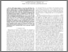# Weighted tradeoff between effective capacity and energy efficiency

Yu, Wenjuan and Musavian, Leila and Ni, Qiang (2015) Weighted tradeoff between effective capacity and energy efficiency. In: Communications (ICC), 2015 IEEE International Conference on. IEEE, GBR, pp. 238-243. ISBN 9781467364324Preview
PDF (2015ICC-Weighted-sum (2))
2015ICC_Weighted_sum_2_.pdf - Accepted Version

## Abstract

This paper proposes a new power allocation technique to jointly optimize link-layer energy efficiency (EE) and effective capacity (EC) of a Rayleigh flat-fading channel with delay-outage probability constraints. Specifically, EE is formulated as the ratio of EC to the sum of transmission power and rate-independent circuit power consumption. A multi-objective optimization problem (MOP) to jointly maximize EE and EC is then formulated. By introducing importance weight into the MOP, we can flexibly change the priority level of EE and EC, and convert the MOP into a single-objective optimization problem (SOP) which can be solved using fractional programming. At first, for a given importance weight and a target delay-outage probability, the optimum average transmission power level to maximize the SOP is found. Then, the optimal power allocation strategy is derived based on the obtained average input power level. Simulation results confirm the analytical derivations and further show the effects of circuit power, importance weight, and transmission power constraint limit on the achievable tradeoff performance.

Item Type:
Contribution in Book/Report/Proceedings